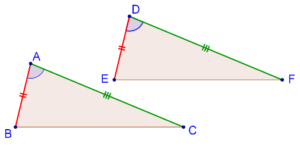# The SAS Criterion

Go back to  'Triangles'

## Introduction to Congruency:

If two triangles are congruent it means that:

• Three sides of one triangle will be (respectively) equal to the three sides of the other
• Three angles of one triangle will be (respectively) equal to the three angles of the other

However, in order to be sure that two triangles are congruent, we do not necessarily need to have information about all sides and all angles.

## What is SAS congruence criterion?

The simplest criterion for the congruence of two triangles is the Side-Angle-Side congruence criterion. This states that if two sides of one triangle, and the angle contained between these two sides, are respectively equal to two sides of another triangle and the angle contained between them, then the two triangles will be congruent. Let us understand this through a diagram.

Consider the following two triangles, $$\Delta ABC$$ and $$\Delta DEF$$:Suppose that,

$AB = DE,$

$AC = DF,$

$\angle A = \angle D$

Now, can you intuitively feel that the two triangles will be congruent? Suppose that you were to try and (exactly) superimpose $$\Delta DEF$$ on $$\Delta ABC$$. You would do this by moving the vertex $$D$$ onto the vertex $$A$$, and aligning one side, say $$DE$$, with $$AB$$. Then, because $$\angle A = \angle D$$, the side $$DF$$ will automatically become exactly aligned with the side $$AC$$, and so $$E$$ would be lying on top of $$B$$, and $$F$$ would be lying on top of $$C$$. This means that $$DF$$ will also be exactly aligned with and equal to $$BC$$.

Thus, you would be able to exactly superimpose $$\Delta DEF$$ on $$\Delta ABC$$ – the two triangles are thus congruent.

Note: The SAS congruence criterion is taken to be an axiom.

grade 9 | Questions Set 2